# How to Compute for the Chi-Square Value and Interpret the Results

Knoji reviews products and up-and-coming brands we think you'll love. In certain cases, we may receive a commission from brands mentioned in our guides. Learn more.
Straightforward tips on how to compute for the chi-square value and interpret the results.

In statistics, one of the most commonly used tests to find out the association between two variables is the Chi-Square test. The Chi-Square test is a very powerful nonparametric test to find out the relationship between two categorical variables. Nonparametric tests are applied when the data are not normally distributed. This test is most appropriate when data are in the form of frequencies.

Originator of the Chi-Square Test

The Chi-Square test is a statistical test devised by Karl Pearson, a notable mathematician who established the discipline of mathematical statistics. The Chi-Square test is just one of the many statistical tools that Karl Pearson developed. Pearson also originated classical statistical tests like the Pearson’s Product Moment Correlation Coefficient and the Principal Component Analysis.

What does Chi-Square Test Do?

The Chi-Square test is used to determine if a null hypothesis stating that the frequency distribution of certain events observed in a sample is in consonance with a particular theoretical distribution. These events must be mutually exclusive, meaning, they do not overlap with each other but are distinctly unique. Gender as a variable, for example, consists of male and female. Males are distinctively different from females. The probability of total events is equal to 1.

The Chi-Square test assesses two types of comparison: 1) tests of goodness of fit, and 2) tests of independence. A test of goodness of fit aims to find out if an observed frequency distribution differs from a theoretical distribution while a test for independence assesses whether paired observations on two variables are independent of or not related with each other. These variables are arranged in a contingency table with a certain number of rows and columns depending on the categories in variables considered.

When is Chi-Square Appropriately Used?

The conditions to be observed for the use of Chi-Square are given below:

1. When data are in the form of frequencies, i.e., they fall within two categorical variables,

2. The data must be independent of each other,

3. When there are more than 40 samples in a 2 x 2 (2 rows and 2 columns) contingency table,

4. One or more of the expected frequencies (computed using the total of rows and columns and the grand total) are not smaller than 5 or a 2 x 2 table or if the table is larger than 2 x 2, 20 percent of the cells have expected frequencies not smaller than 5,

5. No cell should have an expected frequency of lower than 1.

Formula for Chi-Square

The formula for computing the Chi-Square value is given below: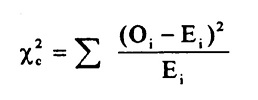where

Oi = observed frequency

Ei = is the expected frequency

Ei = (row total x column total)/grand total

How to Compute for the Value of Chi-Square

A researcher might want to know if there is a significant association between the variables gender and soft drink choice (in this example, Coke and Pepsi were considered). The null hypothesis would be

Ho: There is no significant association between gender and soft drink choice.

Both of these variables are categorical or nominal variables and the Chi-Square test is the most appropriate test. For example, a total of 50 respondents were sampled. The following data including totals for the rows and columns was obtained:To obtain the Chi-Square value, and using the formula above, the results are given below.Notice that the computer Chi-Square value is 6.65. So what is the meaning of this value?

In order to find out if this value is significant or not such that the researcher can conclude whether there is an association between gender and soft drink choice, the tabular Chi-Square value must be obtained. The tabular Chi-Square value can be obtained by choosing the alpha level (denoted by the symbol alpha or) and finding the value of the degree of freedom (df). The standard or most commonly used  level is 0.05. This simply means that you leave open a 5% possibility of being wrong if you reject the null hypothesis. The df, on the other hand, is computed by subtracting 1 from the number of rows and columns and multiplying the difference. There are two rows for gender, and two rows for soft drink choice. In this example, the df is computed thus:

df = (row – 1) x (column – 1)

= (2-1) x (2-1)

= 1 x 1

df = 1

Using the table for Chi-Square below, the corresponding tabular value for df = 1 is equal to 3.84.The association between gender and soft drink choice is significant if the computed Chi-Square value is greater than the tabular value. If not, then the association is not significant. In this example, therefore, the researcher concludes that there is a significant association between gender and soft drink choice because the computed Chi-Square value (6.65) is greater than the tabular value (3.84). Looking at the table of soft drink choice, the researcher can say with confidence that males prefer Coke while the females prefer Pepsi.

©Patrick Regoniel 23 September 2010 The Chi Square Test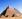Ramz
0
Posted on Sep 27, 2010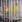Phoenix Montoya
0
Posted on Sep 24, 2010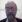Jerry Walch
0
Posted on Sep 24, 2010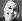Sharla Smith
0
Posted on Sep 23, 2010